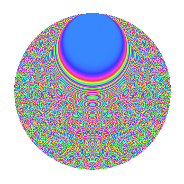# Properties

 Label 3150.2.csLevel 3150 Weight 2 Character orbit cs Rep. character $$\chi_{3150}(211,\cdot)$$ Character field $$\Q(\zeta_{15})$$ Dimension 1440 Sturm bound 1440

# Related objects

## Defining parameters

 Level: $$N$$ = $$3150 = 2 \cdot 3^{2} \cdot 5^{2} \cdot 7$$ Weight: $$k$$ = $$2$$ Character orbit: $$[\chi]$$ = 3150.cs (of order $$15$$ and degree $$8$$) Character conductor: $$\operatorname{cond}(\chi)$$ = $$225$$ Character field: $$\Q(\zeta_{15})$$ Sturm bound: $$1440$$

## Dimensions

The following table gives the dimensions of various subspaces of $$M_{2}(3150, [\chi])$$.

Total New Old
Modular forms 5824 1440 4384
Cusp forms 5696 1440 4256
Eisenstein series 128 0 128

## Trace form

 $$1440q - 8q^{3} + 180q^{4} - 16q^{5} - 8q^{9} + O(q^{10})$$ $$1440q - 8q^{3} + 180q^{4} - 16q^{5} - 8q^{9} - 8q^{11} + 12q^{12} - 8q^{14} - 28q^{15} + 180q^{16} - 8q^{18} + 4q^{20} - 8q^{23} - 48q^{25} + 16q^{27} - 24q^{29} - 16q^{30} + 24q^{31} - 8q^{33} + 16q^{36} - 48q^{37} + 24q^{38} + 12q^{39} + 16q^{44} - 64q^{45} - 52q^{47} - 24q^{48} - 720q^{49} + 4q^{50} - 40q^{51} - 16q^{53} + 48q^{54} - 24q^{55} - 8q^{56} - 48q^{57} - 12q^{59} + 8q^{60} + 12q^{63} - 360q^{64} + 176q^{65} - 36q^{67} + 52q^{69} - 80q^{71} + 16q^{72} + 160q^{74} - 48q^{75} + 112q^{78} - 8q^{80} - 64q^{81} + 96q^{82} + 40q^{83} - 24q^{85} - 40q^{86} - 60q^{87} + 160q^{89} + 20q^{90} + 12q^{92} - 8q^{93} + 28q^{95} - 24q^{97} + 8q^{99} + O(q^{100})$$

## Decomposition of $$S_{2}^{\mathrm{new}}(3150, [\chi])$$ into newform subspaces

The newforms in this space have not yet been added to the LMFDB.

## Decomposition of $$S_{2}^{\mathrm{old}}(3150, [\chi])$$ into lower level spaces

$$S_{2}^{\mathrm{old}}(3150, [\chi]) \cong$$ $$S_{2}^{\mathrm{new}}(225, [\chi])$$$$^{\oplus 4}$$$$\oplus$$$$S_{2}^{\mathrm{new}}(450, [\chi])$$$$^{\oplus 2}$$$$\oplus$$$$S_{2}^{\mathrm{new}}(1575, [\chi])$$$$^{\oplus 2}$$

## Hecke Characteristic Polynomials

There are no characteristic polynomials of Hecke operators in the database